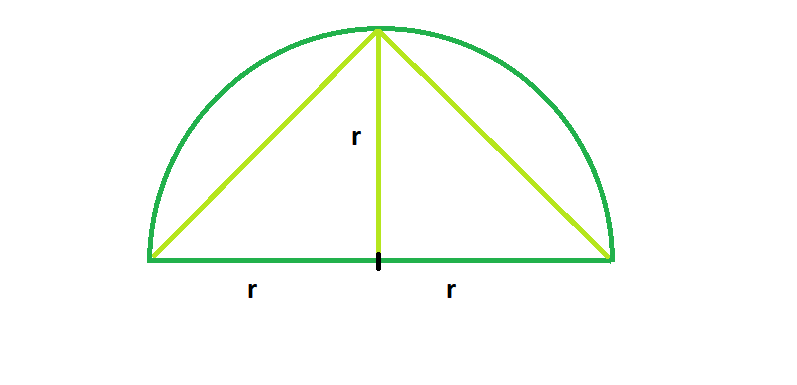# Largest triangle that can be inscribed in a semicircle

Given a semicircle with radius r, we have to find the largest triangle that can be inscribed in the semicircle, with base lying on the diameter.

Examples:

```Input: r = 5
Output: 25

Input: r = 8
Output: 64
```

## Recommended: Please try your approach on {IDE} first, before moving on to the solution.Approach: From the figure, we can clearly understand the biggest triangle that can be inscribed in the semicircle has height r. Also, we know the base has length 2r. So the triangle is an isosceles triangle.

So, Area A: = (base * height)/2 = (2r * r)/2 = r^2

Below is the implementation of above approach:

## C++

 `// C++ Program to find the biggest triangle ` `// which can be inscribed within the semicircle ` `#include ` `using` `namespace` `std; ` ` `  `// Function to find the area ` `// of the triangle ` `float` `trianglearea(``float` `r) ` `{ ` ` `  `    ``// the radius cannot be negative ` `    ``if` `(r < 0) ` `        ``return` `-1; ` ` `  `    ``// area of the triangle ` `     ``return` `r * r; ` `} ` ` `  `// Driver code ` `int` `main() ` `{ ` `    ``float` `r = 5; ` `    ``cout << trianglearea(r) << endl; ` `    ``return` `0; ` `} `

## Java

 `// Java  Program to find the biggest triangle  ` `// which can be inscribed within the semicircle  ` `import` `java.io.*; ` ` `  `class` `GFG { ` `    `  ` `  `// Function to find the area  ` `// of the triangle  ` `static` `float` `trianglearea(``float` `r)  ` `{  ` ` `  `    ``// the radius cannot be negative  ` `    ``if` `(r < ``0``)  ` `        ``return` `-``1``;  ` ` `  `    ``// area of the triangle  ` `    ``return` `r * r;  ` `}  ` ` `  `// Driver code  ` ` `  ` `  `    ``public` `static` `void` `main (String[] args) { ` `        ``float` `r = ``5``;  ` `    ``System.out.println( trianglearea(r));  ` `    ``} ` `} ` `// This code is contributed   ` `// by chandan_jnu. `

## Python 3

 `# Python 3 Program  to find the biggest triangle  ` `# which can be inscribed within the semicircle ` ` `  `# Function to find the area  ` `# of the triangle  ` `def` `trianglearea(r) : ` ` `  `    ``# the radius cannot be negative  ` `    ``if` `r < ``0` `: ` `        ``return` `-``1` ` `  `    ``#  area of the triangle  ` `    ``return` `r ``*` `r ` ` `  ` `  `# Driver Code ` `if` `__name__ ``=``=` `"__main__"` `: ` ` `  `    ``r ``=` `5` `    ``print``(trianglearea(r)) ` ` `  `# This code is contributed by ANKITRAI1 `

## C#

 `// C# Program to find the biggest  ` `// triangle which can be inscribed  ` `// within the semicircle  ` `using` `System;  ` ` `  `class` `GFG  ` `{ ` `     `  `// Function to find the area  ` `// of the triangle  ` `static` `float` `trianglearea(``float` `r)  ` `{  ` ` `  `    ``// the radius cannot be negative  ` `    ``if` `(r < 0)  ` `        ``return` `-1;  ` ` `  `    ``// area of the triangle  ` `    ``return` `r * r;  ` `}  ` ` `  `// Driver code  ` `public` `static` `void` `Main ()  ` `{ ` `    ``float` `r = 5;  ` `    ``Console.Write(trianglearea(r));  ` `} ` `} ` ` `  `// This code is contributed  ` `// by ChitraNayal `

## PHP

 ` `

Output:

```25
```

Attention reader! Don’t stop learning now. Get hold of all the important DSA concepts with the DSA Self Paced Course at a student-friendly price and become industry ready.

My Personal Notes arrow_drop_upBudding Web DeveloperKeen learnerAverage CoderDancer&Social Activist

If you like GeeksforGeeks and would like to contribute, you can also write an article using contribute.geeksforgeeks.org or mail your article to contribute@geeksforgeeks.org. See your article appearing on the GeeksforGeeks main page and help other Geeks.

Please Improve this article if you find anything incorrect by clicking on the "Improve Article" button below.

Article Tags :
Practice Tags :

Be the First to upvote.

Please write to us at contribute@geeksforgeeks.org to report any issue with the above content.